二位的小波变换 图像处理_图像处理小波变换 - CSDN
• //图像小波变换 /* nLayer：变换尺度 type：小波类型 */ void ImageDWT(IplImage* src, IplImage* dst, int nLayer, char* type); //通用的小波变换 /* nLayer:小波变换的层数 type:选取的小波名称 可用为...
编程环境：windows下结合opencv库
//二维图像小波变换
/*
nLayer：变换尺度
type：小波类型
*/
void ImageDWT(IplImage* src, IplImage* dst, int nLayer, char* type);//通用的二维小波变换
/*
nLayer:小波变换的层数
type:选取的小波名称
可用为: "haar","db4","sym4"
使用Mallat算法，并利用傅里叶变换来快速执行卷积运算
src与dst深度均为IPL_DEPTH_8U
*/
void ImageDWT(IplImage* src, IplImage* dst, int nLayer, char* type)
{
if (!src || !dst)
{
return;
}
//判断src与dst的长度宽度是否为2的倍数
if (((dst->width >> nLayer) << nLayer != dst->width)
|| ((dst->height >> nLayer) << nLayer != dst->height)
|| (src->nChannels != 1)
|| (dst->nChannels != 1)
|| (nLayer <= 0))
{
return;
}

CvMat* pMatData = NULL;//用于保存计算过程中的向量
CvMat* pMatCA = NULL;
CvMat* pMatCD = NULL;
CvMat* pMatDst = cvCreateMat(dst->height, dst->width, CV_64FC1);//结果矩阵
CvMat tmp;
int nWidth = dst->width;
int nHeight = dst->height;
int nHalfW = nWidth / 2;
int nHalfH = nHeight / 2;
int i, x, y;
double* *pfDst = (double**)(malloc(sizeof(double*) * pMatDst->height));//pMatDst数据的起始地址
double* pfCA;//CA数据起始地址
double* pfCD;//CD数据起始地址
double* pfData;//Data数据起始地址

//保存图像数据每行的起始地址
for (y = 0; y < nHeight; y++)
{
pfDst[y] = (double*)(pMatDst->data.ptr + y * pMatDst->step);
}

//预先填充pMatDst
cvZero(pMatDst);
cvGetSubRect(pMatDst, &tmp, cvRect(0, 0, src->width, src->height));
cvScale(src, &tmp);

while (nLayer > 0)
{
//行变换
pMatData = cvCreateMat(1, nWidth, CV_64FC1);
pMatCA =  cvCreateMat(1, nHalfW, CV_64FC1);
pMatCD =  cvCreateMat(1, nHalfW, CV_64FC1);
y = 0;
for (y = 0; y < nHeight; y++)
{
pfCA = (double*)(pMatCA->data.ptr);
pfCD = (double*)(pMatCD->data.ptr);
pfData = (double*)(pMatData->data.ptr);
//获取每一行数据
for (i = 0; i < nWidth; i++)
{
pfData[i] = pfDst[y][i];
}
cvDWT(pMatData, pMatCA, pMatCD, type);//对每一行数据进行一维快速小波变换
//将小波变换结果拼接到一起并设置到pMatDst中
for (i = 0; i < nWidth; i++)
{
if (i < nHalfW)
{
pfDst[y][i] = pfCA[i];
}
else
{
pfDst[y][i] = pfCD[i - nHalfW];
}
}
}
cvReleaseMat(&pMatData);
cvReleaseMat(&pMatCA);
cvReleaseMat(&pMatCD);

//列变换
pMatData = cvCreateMat(1, nHeight, CV_64FC1);
pMatCA =  cvCreateMat(1, nHalfH, CV_64FC1);
pMatCD =  cvCreateMat(1, nHalfH, CV_64FC1);
y = 0;
for (x = 0; x < nWidth; x++)
{
pfCA = (double*)(pMatCA->data.ptr);
pfCD = (double*)(pMatCD->data.ptr);
pfData = (double*)(pMatData->data.ptr);
//获取每一列数据
for (i = 0; i < nHeight; i++)
{
pfData[i] = pfDst[i][x];
}
cvDWT(pMatData, pMatCA, pMatCD, type);//对每一列数据进行一维快速小波变换
//将小波变换结果拼接到一起并设置到dst中
for (i = 0; i < nHeight; i++)
{
if (i < nHalfH)
{
pfDst[i][x] = pfCA[i];
}
else
{
pfDst[i][x] = pfCD[i - nHalfH];
}
}
}
cvReleaseMat(&pMatData);
cvReleaseMat(&pMatCA);
cvReleaseMat(&pMatCD);

//一层变换后的宽度长度缩小
nWidth = nHalfW;
nHeight = nHalfH;
nHalfW = nHalfW / 2;
nHalfH = nHalfH / 2;
nLayer--;
}
//缩放以便显示
cvScale(pMatDst, dst);
}
展开全文• 最近在看物体识别论文摘要，好多论文中涉及到使用离散余弦傅里叶变换DFT（Discrete Fourier Transform）对图像进行处理，因此特地看了这部分的内容，傅里叶变换和小波变换。一、DFT的原理：以图像为例，归一化的...
最近在看物体识别论文摘要，好多论文中涉及到使用离散余弦傅里叶变换DFT（Discrete Fourier Transform）对图像进行处理，因此特地看了这部分的内容，傅里叶变换和小波变换。一、DFT的原理：以二维图像为例，归一化的二维离散傅里叶变换可以写成如下形式：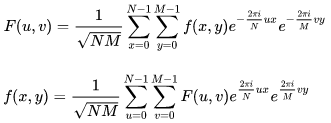其中f(x,y)表示图像的空间域的值，而F表示频域的值，傅里叶转换的结果为复数，这也表明，傅里叶变换其实是一副实数图像和虚数图像叠加或幅度图像和相位图像叠加的结果，在实际的图像处理算法中，仅有幅度图像能够用得到，因为其包含了图像的所有几何结构信息。但是如果想通过修改幅度图和相位图来修改原空间图像，需保留幅度图和相位图来进行傅里叶变换，从而得到修改后的图像。在频域里面，高频部分代表了图像边缘、线条以及纹理等细节信息，低频部分代表了图像的轮廓信息。在这里首先介绍下空间域和频率域：空间域：一般情况下，空间域的图像为f(x,y),形象一点就是一个二维矩阵，每个坐标对应一个颜色值。频率域：频率：对于图像来说，可以指图像颜色值的梯度，即灰度级的变化速度。幅度：频率的权，即该频率所占的比例。二、代码实现与效果#include<iostream>
#include<opencv2/core/core.hpp>
#include<opencv2/highgui/highgui.hpp>
#include<opencv2/imgproc/imgproc.hpp>
using namespace std;
using namespace cv;
int main()
{
//(1)读取原图像
if (!src.data)
{
cout << "Reading image error!" << endl;
return false;
}
imshow("src",src);
//（2）将输入图像扩展到最佳尺寸，将添加的像素扩展为0
int m = getOptimalDFTSize(src.rows);
int n = getOptimalDFTSize(src.cols);
//(3)为傅里叶变换的结果（实部和虚部）分配存储空间
//将planes数组合并成一个多通道的数组complexI
Mat complexI;
merge(planes,2,complexI);
//(4)离散傅里叶变换
dft(complexI,complexI);
//(5)将复数转换为幅值，即=> log(1 + sqrt(Re(DFT(I))^2 + Im(DFT(I))^2))
split(complexI, planes); // 将多通道数组complexI分离成几个单通道数组，planes = Re(DFT(I), planes = Im(DFT(I))
magnitude(planes, planes, planes);//planes = magnitude
Mat magnitudeImage = planes;
//(5)进行对数尺度(logarithmic scale)缩放
//由于幅度范围太大，不适合在屏幕显示。为了在屏幕显示，用对数尺度来替换线性尺度M1=log(1+M)
magnitudeImage += Scalar::all(1);//转换到对数尺度
log(magnitudeImage, magnitudeImage);//求自然对数
//(6)剪切和重分布幅度图象限
/*
剔除第二步添加的像素。重分布是把四个象限的四张图像拼接到一起
*/
//若有奇数行或奇数列，进行频谱裁剪
magnitudeImage = magnitudeImage(Rect(0, 0, magnitudeImage.cols & -2, magnitudeImage.rows & -2));
//重新排列傅立叶图像中的象限，使得原点位于图像中心
int cx = magnitudeImage.cols / 2;
int cy = magnitudeImage.rows / 2;
Mat q0(magnitudeImage, Rect(0, 0, cx, cy));  //roi区域的左上
Mat q1(magnitudeImage, Rect(cx, 0, cx, cy));  //roi区域的右上
Mat q2(magnitudeImage, Rect(0, cy, cx, cy));  //roi区域的左下
Mat q3(magnitudeImage, Rect(cx, cy, cx, cy)); //roi区域的右下
//交换象限（左上与右下进行交换）
Mat tmp;
q0.copyTo(tmp);
q3.copyTo(q0);
tmp.copyTo(q3);
//交换象限（右上与左下进行交换）
q1.copyTo(tmp);
q2.copyTo(q1);
tmp.copyTo(q2);
//(7)归一化，用0到1之间的浮点值将矩阵变换为可视的图像格式
/*
幅度值仍然超过可显示范围[0,1],normalize()归一化后可以显示
*/
normalize(magnitudeImage, magnitudeImage, 0, 1, NORM_MINMAX);
//【9】显示效果图
imshow("dft", magnitudeImage);
//逆变换
Mat _complexim;
complexI.copyTo(_complexim);//把变换结果复制一份，进行逆变换，也就是恢复原图
Mat iDft[] = { Mat::zeros(planes.size(), CV_32F), Mat::zeros(planes.size(), CV_32F) };//创建两个通道，类型为float，大小为填充后的尺寸
idft(_complexim, _complexim);//傅立叶逆变换
split(_complexim, iDft);//结果貌似也是复数
magnitude(iDft, iDft, iDft);//分离通道，主要获取0通道
normalize(iDft, iDft, 1, 0, CV_MINMAX);//归一化处理，float类型的显示范围为0-1,大于1为白色，小于0为黑色
imshow("idft",iDft);
waitKey(0);
return 0;
}实现结果：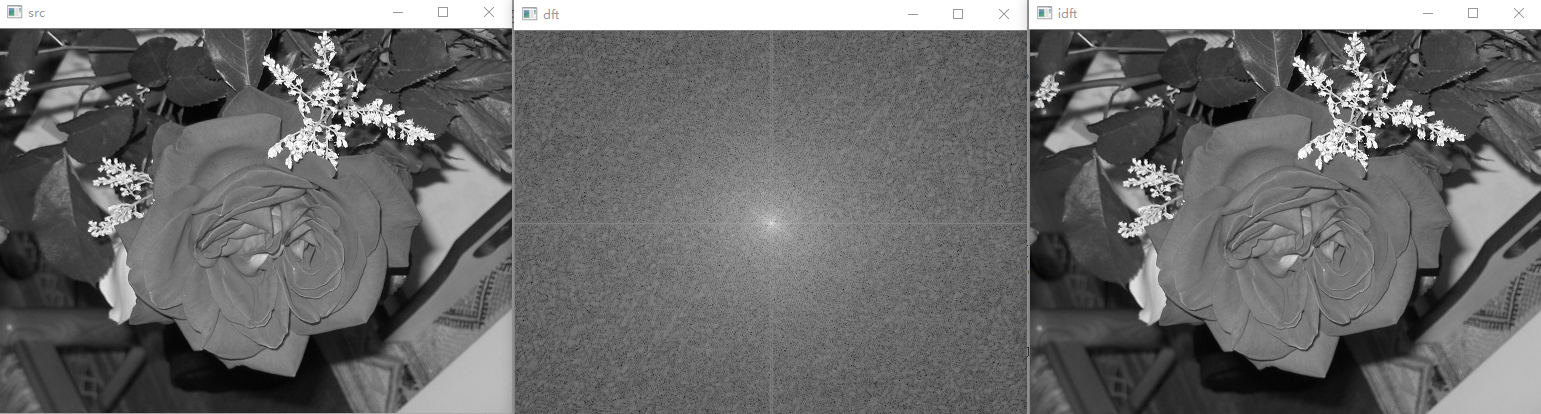二、小波变换# include<opencv2/opencv.hpp>
# include<iostream>

using namespace std;
using namespace cv;

int main()
{
int Height = img.cols;
int Width = img.rows;
int depth = 1;    //定义分解深度,也就是几级分解
int depthcount = 1;
Mat tmp = Mat::ones(Width, Height, CV_32FC1);//CV_32FC1表示32位float，这在32位编译器上是32位float，也就是单精度。CV_64FC1在32位编译器上是64位float，也就是双精度。
Mat wavelet = Mat::ones(Width, Height, CV_32FC1);
Mat imgtmp = img.clone();
imgtmp.convertTo(imgtmp, CV_32FC1);
while (depthcount <= depth){
Width = img.rows / depthcount;
Height = img.cols / depthcount;
for (int i = 0; i < Width; i++)
{
for (int j = 0; j < Height / 2; j++)
{
tmp.at<float>(i, j) = (imgtmp.at<float>(i, 2 * j) + imgtmp.at<float>(i, 2 * j + 1)) / 2;//整体信息
tmp.at<float>(i, j + Height / 2) = (imgtmp.at<float>(i, 2 * j) - imgtmp.at<float>(i, 2 * j + 1)) / 2;//细节信息
}
}
for (int i = 0; i < Width / 2; i++)
{
for (int j = 0; j < Height; j++)
{
wavelet.at<float>(i, j) = (tmp.at<float>(2 * i, j) + tmp.at<float>(2 * i + 1, j)) / 2;//整体信息
wavelet.at<float>(i + Width / 2, j) = (tmp.at<float>(2 * i, j) - tmp.at<float>(2 * i + 1, j)) / 2;//细节信息
}
}
imgtmp = wavelet;
depthcount++;
}
wavelet.convertTo(wavelet, CV_8UC1);
wavelet += 100;            //图像暗度过低，所以这里我加了50
imshow("小波变换", wavelet);
waitKey(0);
return 0;
}实现效果：.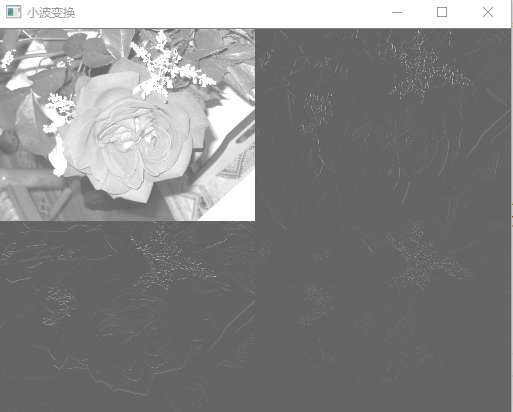展开全文离散傅里叶变换 小波变换
• 3. 图像小波变换的 Matlab 实现 3.1 一维小波变换的 Matlab 实现 (1) dwt 函数 功能：一维离散小波变换 格式：[cA,cD]=dwt(X,'wname')  [cA,cD]=dwt(X,Lo_D,Hi_D) 说明：[cA,cD]=dwt(X,'wname') 使用指定...
3. 图像小波变换的
Matlab 实现

3.1 一维小波变换的 Matlab 实现
(1) dwt 函数
功能：一维离散小波变换
格式：[cA,cD]=dwt(X,'wname')
[cA,cD]=dwt(X,Lo_D,Hi_D)
说明：[cA,cD]=dwt(X,'wname') 使用指定的小波基函数 'wname' 对信号 X 进行分解，cA、cD 分别为近似分量和细节分量；[cA,cD]=dwt(X,Lo_D,Hi_D) 使用指定的滤波器组 Lo_D、Hi_D 对信号进行分解。
(2) idwt 函数
功能：一维离散小波反变换
格式：X=idwt(cA,cD,'wname')
X=idwt(cA,cD,Lo_R,Hi_R)
X=idwt(cA,cD,'wname',L)
X=idwt(cA,cD,Lo_R,Hi_R,L)
说明：X=idwt(cA,cD,'wname') 由近似分量 cA 和细节分量 cD 经小波反变换重构原始信号 X 。
'wname' 为所选的小波函数
X=idwt(cA,cD,Lo_R,Hi_R) 用指定的重构滤波器 Lo_R 和 Hi_R 经小波反变换重构原始信号 X 。
X=idwt(cA,cD,'wname',L) 和 X=idwt(cA,cD,Lo_R,Hi_R,L) 指定返回信号 X 中心附近的 L 个点。

3.2 二维小波变换的 Matlab 实现

二维小波变换的函数
-------------------------------------------------
函数名                 函数功能
---------------------------------------------------
dwt2             二维离散小波变换
wavedec2       二维信号的多层小波分解
idwt2           二维离散小波反变换
waverec2         二维信号的多层小波重构
wrcoef2           由多层小波分解重构某一层的分解信号
upcoef2           由多层小波分解重构近似分量或细节分量
detcoef2         提取二维信号小波分解的细节分量
appcoef2         提取二维信号小波分解的近似分量
upwlev2         二维小波分解的单层重构
dwtpet2         二维周期小波变换
idwtper2         二维周期小波反变换
-------------------------------------------------------------

(1) wcodemat 函数
功能：对数据矩阵进行伪彩色编码
格式：Y=wcodemat(X,NB,OPT,ABSOL)
Y=wcodemat(X,NB,OPT)
Y=wcodemat(X,NB)
Y=wcodemat(X)
说明：Y=wcodemat(X,NB,OPT,ABSOL) 返回数据矩阵 X 的编码矩阵 Y ；NB 伪编码的最大值，即编码范围为 0～NB，缺省值 NB＝16；
OPT 指定了编码的方式（缺省值为 'mat'），即：
OPT＝'row' ，按行编码
OPT＝'col' ，按列编码
OPT＝'mat' ，按整个矩阵编码
ABSOL 是函数的控制参数（缺省值为 '1'），即：
ABSOL＝0 时，返回编码矩阵
ABSOL＝1 时，返回数据矩阵的绝对值 ABS(X)

(2) dwt2 函数
功能：二维离散小波变换
格式：[cA,cH,cV,cD]=dwt2(X,'wname')
[cA,cH,cV,cD]=dwt2(X,Lo_D,Hi_D)
说明：[cA,cH,cV,cD]=dwt2(X,'wname')使用指定的小波基函数 'wname' 对二维信号 X 进行二维离散小波变幻；cA，cH,cV,cD 分别为近似分量、水平细节分量、垂直细节分量和对角细节分量；[cA,cH,cV,cD]=dwt2(X,Lo_D,Hi_D) 使用指定的分解低通和高通滤波器 Lo_D 和 Hi_D 分解信号 X 。

(3) wavedec2 函数
功能：二维信号的多层小波分解
格式：[C,S]=wavedec2(X,N,'wname')
[C,S]=wavedec2(X,N,Lo_D,Hi_D)
说明：[C,S]=wavedec2(X,N,'wname') 使用小波基函数 'wname' 对二维信号 X 进行 N 层分解；[C,S]=wavedec2(X,N,Lo_D,Hi_D) 使用指定的分解低通和高通滤波器 Lo_D 和 Hi_D 分解信号 X 。

(4) idwt2 函数
功能：二维离散小波反变换
格式：X=idwt2(cA,cH,cV,cD,'wname')
X=idwt2(cA,cH,cV,cD,Lo_R,Hi_R)
X=idwt2(cA,cH,cV,cD,'wname',S)
X=idwt2(cA,cH,cV,cD,Lo_R,Hi_R,S)
说明：X=idwt2(cA,cH,cV,cD,'wname') 由信号小波分解的近似信号 cA 和细节信号 cH、cH、cV、cD 经小波反变换重构原信号 X ；X=idwt2(cA,cH,cV,cD,Lo_R,Hi_R) 使用指定的重构低通和高通滤波器 Lo_R 和 Hi_R 重构原信号 X ；X=idwt2(cA,cH,cV,cD,'wname',S) 和 X=idwt2(cA,cH,cV,cD,Lo_R,Hi_R,S) 返回中心附近的 S 个数据点。

(5) waverec2 函数
说明：二维信号的多层小波重构
格式：X=waverec2(C,S,'wname')
X=waverec2(C,S,Lo_R,Hi_R)
说明：X=waverec2(C,S,'wname') 由多层二维小波分解的结果 C、S 重构原始信号 X ，'wname' 为使用的小波基函数；X=waverec2(C,S,Lo_R,Hi_R) 使用重构低通和高通滤波器 Lo_R 和 Hi_R 重构原信号。
图像处理工具箱1. 图像和图像数据
缺省情况下，MATLAB将图像中的数据存储为双精度类型(double)，64位浮点
数，所需存储量很大；MATLAB还支持另一种类型无符号整型(uint8)，即图像矩
阵中每个数据占用1个字节。
在使用MATLAB工具箱时，一定要注意函数所要求的参数类型。另外，uint8
与double两种类型数据的值域不同，编程需注意值域转换。
从uint8到double的转换
---------------------------------------------
图像类型         MATLAB语句
---------------------------------------------
索引色             B=double(A)+1
索引色或真彩色 B=double(A)/255
二值图像           B=double(A)
---------------------------------------------

从double到uint8的转换
---------------------------------------------
图像类型         MATLAB语句
---------------------------------------------
索引色               B=uint8(round(A-1))
索引色或真彩色     B=uint8(round(A*255))
二值图像             B=logical(uint8(round(A)))
---------------------------------------------

2. 图像处理工具箱所支持的图像类型

2.1 真彩色图像
R、G、B三个分量表示一个像素的颜色。如果要读取图像中(100,50)处的像素值，
可查看三元数据(100,50,1:3)。
真彩色图像可用双精度存储，亮度值范围是[0,1]；比较符合习惯的存储方法是用无
符号整型存储，亮度值范围[0,255]

2.2 索引色图像
包含两个结构，一个是调色板，另一个是图像数据矩阵。调色板是一个有3列和若干行
的色彩映象矩阵，矩阵每行代表一种颜色，3列分别代表红、绿、蓝色强度的双精度数。

注意：MATLAB中调色板色彩强度[0,1]，0代表最暗，1代表最亮。
常用颜色的RGB值
--------------------------------------------
颜色     R   G   B       颜色     R   G   B
--------------------------------------------
黑     0   0   1       洋红     1   0   1
白     1   1   1       青蓝     0   1   1
红     1   0   0       天蓝 0.67 0   1
绿     0   1   0       橘黄     1 0.5 0
蓝     0   0   1       深红   0.5 0   0
黄     1   1   0       灰     0.5 0.5 0.5
--------------------------------------------
产生标准调色板的函数
-------------------------------------------------
函数名       调色板
-------------------------------------------------
Hsv       色彩饱和度，以红色开始，并以红色结束
Hot       黑色－红色－黄色－白色
Cool     
展开全文图像处理
• 兹于2017年12月，应《多媒体技术基础》课程实验的要求，本人就基于维哈尔小波变换算法做了较为深入的理解，用MATLAB和C++语言实现维哈尔小波变换算法。维哈尔小波变换算法一、实验目的掌握小波及小波变换相关...
兹于2017年12月，应《多媒体技术基础》课程实验的要求，本人就基于二维哈尔小波变换算法做了较为深入的理解，用MATLAB和C++语言实现二维哈尔小波变换算法。二维哈尔小波变换算法一、实验目的掌握小波及小波变换相关算法。 二、实验设备与环境Windows 7，MATLAB，DEV C++ 三、实验内容、程序清单及运行结果实验要求根据课本内容，实现图像小波变换算法。具体可参考《多媒体技术基础（第三版）》，可以在这里下载PDF电子书小波与小波变换算法概述小波是定义在有限间隔而且其平均值为零的一种函数。小波变换算法求有限信号的均值和差值：例如：假设有一幅分辨率只有4个像素p0、p1、p2、p3 的一维图像，对应的像素值或者叫做图像位置的系数分别为：[ 9   7     3     5 ]计算它的哈尔小波变换系数。计算步骤如下：步骤1：求均值(averaging)。计算相邻像素对的平均值，得到一幅分辨率比较低的新图像，它的像素数目变成了2个，即新的图像的分辨率是原来的1/2，相应的像素值为：[ 8       4 ]步骤2：求差值(differencing)。很明显，用2个像系表示这幅图像时，图像的信息已经部分丢失。为了能够从由2个像素组成的图像重构出由4个像素组成的原始图像，就需变存储一些图像的细节系数(detail coefficient)，以便在重构时找回丢失的信息。方法是把像素对的第一个像素值减去这个像索对的平均值.或者使用这个像素对的差值除以2。在这个例子中，第一个细节系数是(9-8)=1，因为计算得到的平均值是8，它比9小1面比7大1，存储这个细节系数就可以恢复原始图像的前两个像素值。使用同样的方法，第二个细节系数是(3-4)=-1，存储这个细节系数就可以恢复后2个像素值。因此，原始图像就可以用下面的两个平均值和两个细节系数表示：[ 8       4     1     -1 ]步骤3：重复步骤1和2，把由第一步分解得到的图像进一步分解成分辨率更低的图像和细节系数。在这个例子中，分解到最后，就用一个像素的平均值6和三个细节系数2、1和-1表示整幅图像：[ 6       2     1     -1 ]这个分解过程见下表：    分辨率    平均值     细节系数     4      [ 9   7     3     5 ]       2[ 8   4 ][ 1   -1 ]1[ 6 ][ 2 ]由此可见，通过上述分解就把由4个像素组成的一幅图像用一个平均像素值和3个细节系数表示，这个过程就叫做哈尔小波变换( Haar wavelet transform)，也称哈尔小波分解(Haarwavelet decomposition)。这个概念可以推广到使用其他小波基的变换。从这个例子中我们可以看到：（1）变换过程中没有丢失信息，因为能够从所记录的数据中重构出原始图像。（2）对这个给定的变换，我们可以从所记录的数据中重构出各种分辨率的图像。例如，在分辨率为l的图像基础上重构出分辨率为2的图像，在分辨率为2的图像基础上重构出分辨率为4的图像。（3）通过变换之后产生的细节系数的幅度值比较小，这就为图像压缩提供了一种途径。例如，去掉一些微不足道的细节系数并不影响对重构图像的理解。小波与小波变换算法步骤及核心程序二维哈尔小波变换是将一个由8*8像素组成的图像块的矩阵，进行小波变换时，对矩阵中的每一行进行行变换，然后对行变换后的矩阵每一列进行列变换，最后将变换后的矩阵进行编码。其次可通过设置阈值δ，例如δ≤5，表示把绝对值小于5的细节系数当作“0”看待，从而对编码后的矩阵进行压缩。最后通过逆变换重构源图像矩阵的近似矩阵。 MATLAB代码：% % 使用线性代数方法计算哈尔小波变换
disp '设第1次相乘的矩阵为M1，第2次为M2，第3次为M3。W=M1*M2*M3：'
W = [1/8,1/8,1/4,0,1/2,0,0,0
1/8,1/8,1/4,0,-1/2,0,0,0
1/8,1/8,-1/4,0,0,1/2,0,0
1/8,1/8,-1/4,0,0,-1/2,0,0
1/8,-1/8,0,1/4,0,0,1/2,0
1/8,-1/8,0,1/4,0,0,-1/2,0
1/8,-1/8,0,-1/4,0,0,0,1/2
1/8,-1/8,0,-1/4,0,0,0,-1/2]

disp '源图像的一个图像块矩阵：'
A = [64,2,3,61,60,6,7,57
9,55,54,12,13,51,50,16
17,47,46,20,21,43,42,24
40,26,27,37,36,30,31,33
32,34,35,29,28,38,39,25
41,23,22,44,45,19,18,48
49,15,14,52,53,11,10,56
8,58,59,5,4,62,63,1]

disp '求均值差值后的矩阵：'
T = W'*A*W

% %  设阈值为5，绝对值小于等于5的元素设为0
for i=1:8
for j=1:8
if(abs(T(i,j))<=5)
T(i,j)=0;
end
end
end

disp '设置阈值变换后的矩阵：'
T

disp '逆变换重构后的近似矩阵：'
LA = ((W')^(-1))*T*W^(-1)
运行结果：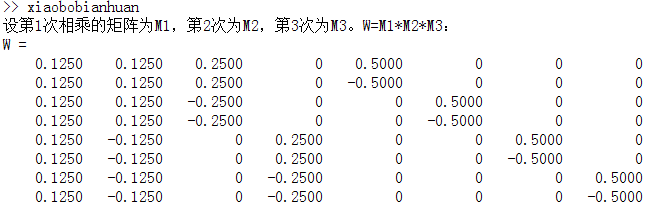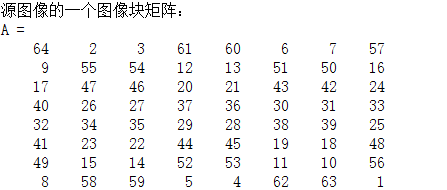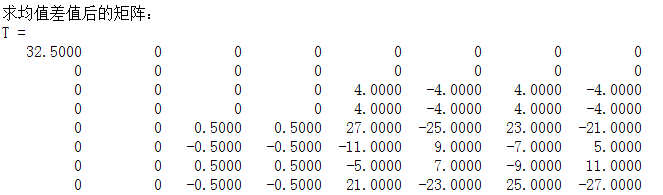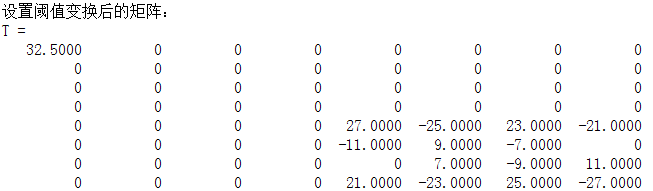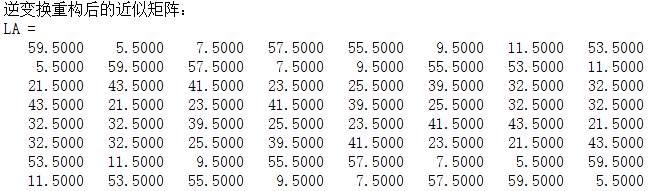C++代码：#include<iostream>
#include<cmath>
#define N 8 //把图像划分成8*8个图像块
using namespace std;
/*使用线性代数方法计算哈尔小波变换*/

/*矩阵转置函数*/
bool  MatrixTranspose(const double source[N][N],double transpose[N][N]){
for(int i=0;i<N;i++){
for(int j=0;j<N;j++){
transpose[i][j] = source[j][i];
}
}
return true;
}

/*矩阵乘法*/
bool MatrixMultiplication(const double operand1[N][N], const double operand2[N][N], double result[N][N]){
for(int i=0;i<N;i++){
for(int j=0;j<N;j++){
double sum=0.0;
for(int k=0;k<N;k++){
sum = sum + operand1[i][k]*operand2[k][j];
}
result[i][j] = sum;
}
}
return true;
}

int main(){
/*设第1次相乘的矩阵为M1，第2次为M2，第3次为M3。W=M1*M2*M3*/
double W[N][N] = {1.0/8,1.0/8,1.0/4,0,1.0/2,0,0,0,
1.0/8,1.0/8,1.0/4,0,-1.0/2,0,0,0,
1.0/8,1.0/8,-1.0/4,0,0,1.0/2,0,0,
1.0/8,1.0/8,-1.0/4,0,0,-1.0/2,0,0,
1.0/8,-1.0/8,0,1.0/4,0,0,1.0/2,0,
1.0/8,-1.0/8,0,1.0/4,0,0,-1.0/2,0,
1.0/8,-1.0/8,0,-1.0/4,0,0,0,1.0/2,
1.0/8,-1.0/8,0,-1.0/4,0,0,0,-1.0/2};

cout<<"设第1次相乘的矩阵为M1，第2次为M2，第3次为M3。W=M1*M2*M3，W矩阵:"<<endl;
for(int i=0;i<N;i++){
for(int j=0;j<N;j++){
cout<<W[i][j]<<"\t";
}
cout<<endl;
}

/*源图像的一个图像块矩阵*/
double A[N][N] = {64,2,3,61,60,6,7,57,
9,55,54,12,13,51,50,16,
17,47,46,20,21,43,42,24,
40,26,27,37,36,30,31,33,
32,34,35,29,28,38,39,25,
41,23,22,44,45,19,18,48,
49,15,14,52,53,11,10,56,
8,58,59,5,4,62,63,1};

cout<<"源图像的一个图像块矩阵:"<<endl;
for(int i=0;i<N;i++){
for(int j=0;j<N;j++){
cout<<A[i][j]<<"\t";
}
cout<<endl;
}

/*矩阵W的转置矩阵*/
double WT[N][N];
MatrixTranspose(W,WT);

/*求均值差值后的矩阵 T = W'*A*W */
double T[N][N];
double temp[N][N];
MatrixMultiplication(WT,A,temp);// temp = W'*A
MatrixMultiplication(temp,W,T);// T = temp*W

cout<<"求均值差值后的矩阵:"<<endl;
for(int i=0;i<N;i++){
for(int j=0;j<N;j++){
cout<<T[i][j]<<"\t";
}
cout<<endl;
}

/*设阈值为5，绝对值小于等于5的元素设为0 */
for(int i=0;i<N;i++){
for(int j=0;j<N;j++){
if(abs(T[i][j])<=5){
T[i][j] = 0.0;
}
}
}

cout<<"设置阈值变换后的矩阵:"<<endl;
for(int i=0;i<N;i++){
for(int j=0;j<N;j++){
cout<<T[i][j]<<"\t";
}
cout<<endl;
}

/*本来想通过用逆变换式子直接用数学公式 A = (W')^(-1)*T*W'  但是C++没有求逆矩阵的库函数，自己编写又太长，故而使用下面这照片这种模拟人工运算方法 */

double tem[N];//该数组作为每一次由均值和差值计算出的结果
/*列逆变换*/
for(int j=0;j<N;j++){
for(int k=0;k<3;k++){//每列8个元素，需要循环3次均值和差值计算
int t = pow(2,k);//t为每次运算结束的位置
for(int i=0;i<t;i++){
tem[2*i] = T[i][j]+T[i+t][j];	//运算得到结果的第一个
tem[2*i+1] = T[i][j]-T[i+t][j]; //运算得到结果的第二个
}
for(int i=0;i<t;i++){				//一次循环完成后要更新T矩阵，因为下次循环要基于上一次循环的结果，但是为什么不在上面那个循环里面不直接赋值给T，不用tem呢，因为上面循环赋值会导致上面运算后续的一些运算的值被覆盖导致错误结果
T[2*i][j] = tem[2*i];
T[2*i+1][j] = tem[2*i+1];
}
}
}

cout<<"列逆变换后的矩阵:"<<endl;
for(int i=0;i<N;i++){
for(int j=0;j<N;j++){
cout<<T[i][j]<<"\t";
}
cout<<endl;
}

/*行逆变换*/
//原理与列逆变换一样
for(int i=0;i<N;i++){
for(int k=0;k<3;k++){//循环3次
int t = pow(2,k);
for(int j=0;j<t;j++){
tem[2*j] = T[i][j]+T[i][j+t];
tem[2*j+1] = T[i][j]-T[i][j+t];
}
for(int j=0;j<t;j++){
T[i][2*j] = tem[2*j];
T[i][2*j+1] = tem[2*j+1];
}
}
}

cout<<"行逆变换,得到重构后的近似矩阵:"<<endl;
for(int i=0;i<N;i++){
for(int j=0;j<N;j++){
cout<<T[i][j]<<"\t";
}
cout<<endl;
}

return 0;
} 运行结果：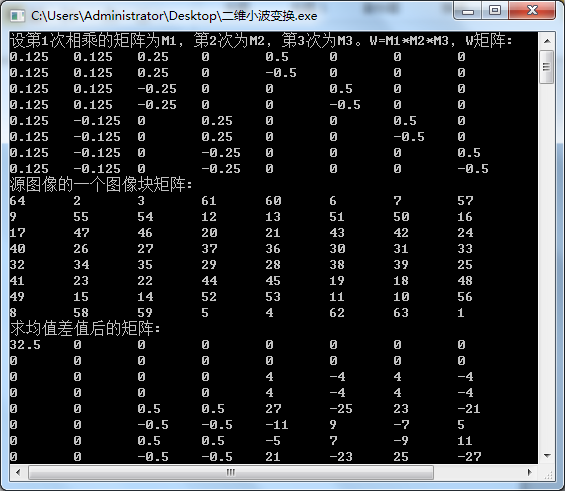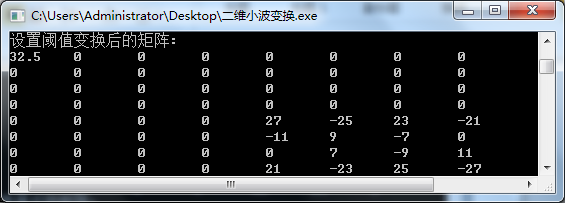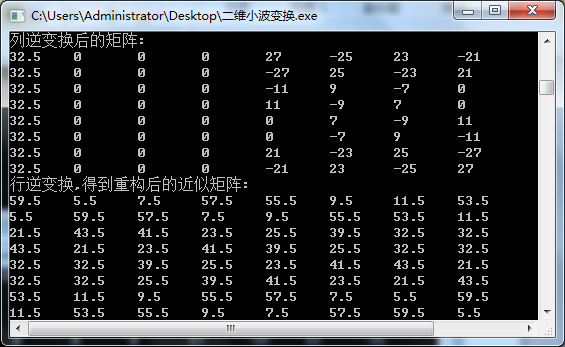四、实验结论、实验体会    本次实验的算法思路很清晰、也比较简单。但是这是相对于来说的，假如是用MATLAB编程，则只要几分钟就能完成这次的实验，因为MATLAB是强大的矩阵处理语言，有非常多的系统类库进行调用，而且语法非常简单。而用C/C++则是非常的麻烦，所有的细节都要自己编写，而且每个细节都要编好长的一段，编码时非常地痛苦，花了很长时间去实现。在进行逆变换时，要用到求解逆矩阵，而求逆矩阵C/C++却没有类库，需要大量的编码工作，所以我选择通过模拟人工计算方式进行逆变换，在这个模拟过程中需要不断地计算每一时刻计算所处的位置、值、和所要存放的位置，存放时是否会跟其它运算是否冲突造成值覆盖等等，非常地麻烦。将整个算法在脑子里一步一步地演进找出所有可能的错误，然后在VS2010进行DEBUG跟踪程序执行，最终完成了此次实验。    这次实验让我更深刻地理解使用数学方法对算法进行精简不仅可以减轻编程人员的负担，同时也能简化计算，提高效率。写得不是很好，望各位大神多多指正，不喜勿喷。
展开全文小波变换 多媒体技术 MATLAB C
• MATLAB 之 图像小波变换函数 1 一维小波变换的 Matlab 实现 (1) dwt 函数 功能：一维离散小波变换 格式：[cA,cD]=dwt(X,'wname')  [cA,cD]=dwt(X,Lo_D,Hi_D) 说明：[cA,cD]=dwt(X,'wname') 使用指定的...matlab 函数 图像处理
• 申明：小波变换 完美通俗解读，是《小波变换和motion信号处理》系列...《小波变换和motion信号处理》系列共包含三篇： 第一篇：基础普及（小波变换完美通俗解读） 第篇：深入小波 第三篇：小波应用限于篇幅关系...小波变换
• 申明：小波变换 完美通俗解读，是《小波变换和motion信号处理》系列中的第一篇。 原始出处为​windstorm的网站http://www.kunli.info/，并非本站原创，但这大师深入浅出的讲解了小波变换，是你在学习小波的过程中...
• 、一维连续小波变换声明： 该文为本人对小波的理解，不保证正确性与严谨性。参考： 《数字图像处理》 Gonzalez P3091. 概述在给定小波函数下，函数f(x)可以由不同尺度和位置的小波函数组合而成，即连续小波逆变换...图像处理
• 下面来介绍一下如何在MATLAB实现CWT，也就是我们常说的连续小波变换，具体如下： 所谓小波(wavelet)，即存在于一个较小区域的波。小波函数的数学定义是：设ψ(t)为一平方可积函数，即ψ(t)∈L2(R)，若其傅里叶变换...MATLAB 连续小波变换
• 3. 图像小波变换的 Matlab 实现3.1 一维小波变换的 Matlab 实现(1) dwt 函数功能：一维离散小波变换格式：[cA,cD]=dwt(X,'wname') [cA,cD]=dwt(X,Lo_D,Hi_D)说明：[cA,cD]=dwt(X,'wname') 使用指定的小波基函数 '...图像处理
• 如果有人还问我，如果第一代小波变换没学好，能否学好第小波变换。答案依然是否定的。但若你问我，没学好傅里叶变换，能否操作（编程）小波变换，或是没学好第一代小波，能否操作二代小波变换，答案是肯定的。 ...傅里叶变换
• 原文地址：【转】小波图像分解与重构作者：freedesert小波图像融合综述（1）分类： 小波变换2007-11-14 11:15 4728人阅读 评论(6) 收藏 举报 图像融合是将两幅或多幅图像融合在一起，以获取对同一场景的更为...
• 近来兴起的数据密集的基于多媒体的Web应用不但需要信号和图像的更有效的编码方式，而且要求对这些信号做基于 存储和通信技术的压缩。静止图像的压缩，已经有ISO（国际标准化组织）和IEC（国际电工技术委员会）建立...小波变换 压缩
• % 此示意程序用DWT实现小波变换 %%%%%%%%%%%%%%%%%%%%%%%%%%%% %%%%%%%%%%%%%%%%%%%%%%%%%%% clear;clc; T=256; % 图像维数 SUB_T=T/2; % 子图维数 %%%%%%%%%%%%%%%%%%%%%%%%%%%%%%%%% %%%%%%%%%%%%%%Matlab
• 本文是边学习边总结和摘抄各参考文献内容而成的，是一篇综述性入门文档，重点在于梳理傅里叶变换到伽柏变换再到小波变换的前因后果，对于一些概念但求多而全，所以可能会有些理解的不准确，后续计划分别再展开学习...傅里叶变换 小波变换 Gabor变换
• 小波变换详解 1，简介 We can use the Fourier Transform to transform a signal from its time-domain to its frequency domain. The peaks in the frequency spectrum indicate the most occurring frequencies...信号 小波变换 神经网络
• 我记的最清楚的是一个这样的问题：试图使用维多尺度离散小波变换，然后对得到的小波系数进行Arnold置乱处理，但是在对处理后的系数进行重构时遇到了困难。利用matlab子带的wavedec2，可以很容易实现分解。格式为：...wavedec2函数 matlab
• 本文是边学习边总结和摘抄各参考文献内容而成的，是一篇综述性入门文档，重点在于梳理傅里叶变换到伽柏变换再到小波变换的前因后果，对于一些概念但求多而全，所以可能会有些理解的不准确，后续计划分别再展开学习...
• 本文为数盟原创译文，转载请注明出处为数盟社区。 作者：DMITRY PETROV 过去的几个周末，Kaggle的这个...在这个项目的竞争中，许多玩家使用图像的哈希值而不是优化模型建立过程中的实际图像。 我发现有趣的是python github 机器学习 算法 优化
• 1.理解维傅里叶变换的定义 ...一个图像尺寸为M×N的 函数的离散傅里叶变换由以下等式给出： 其中 和。其中变量u和v用于确定它们的频率，频域系统是由所张成的坐标系，其中和用做（频率）变量...
...